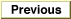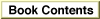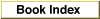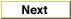# Legacy DocumentImportant: The information in this document is obsolete and should not be used for new development.

Inside Macintosh: QuickDraw GX Environment and Utilities /
Chapter 8 - QuickDraw GX Mathematics / Using QuickDraw GX Mathematics

## Generating Random Numbers

You can use the QuickDraw GX random number functions to return a sequence of random numbers. The `RandomBits` function generates random integers in the range of 0 to 2count - 1, where count is the number of bits in the integer to be generated by the random number generator.

The `SetRandomSeed` function allows you to use a seed other than the default seed. If the `SetRandomSeed` function is not used, the initial seed will always be 0. You can use the `GetRandomSeed` function to return the value of the current seed.

Listing 8-8 is a sample function that generates an unsigned random number between zero and the value passed in the `limit` parameter. It uses the `RandomBits` function, and it also uses the `WideMultiply` function, correcting for the fact that `WideMultiply` works with signed long integers whereas this random generator uses unsigned longs.

Listing 8-8 A random number generator

```unsigned long RandomLong(unsigned long limit)
{
wide temp;
unsigned long random = RandomBits(32, 0);

/* This treats random and limit as signed */
WideMultiply(random, limit, &temp);
if ((long)limit < 0)
temp.hi += random;   /* correct for the "sign" of limit */
if ((long)random < 0)
temp.hi += limit;    /* correct for the "sign" of random */
return temp.hi;
}
```
The general topic of random numbers and the functions you use to generate them generation are discussed in the section "Random Number Generation" beginning on page 8-58.